Author: Oscar Cronquist Article last updated on January 13, 2023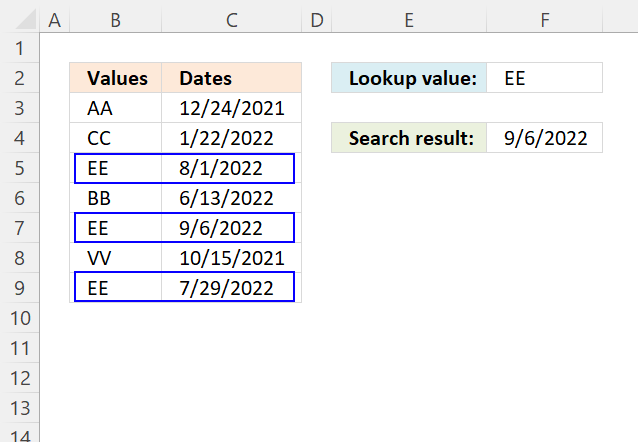This article demonstrates how to return the latest date based on a condition using formulas or a Pivot Table. The condition is specified in cell F2 and the result is in cell F4.

For example, the condition is met in cells B5, B7, and B9. The corresponding dates in column C on the same row are 8/1/2022, 9/6/2022, and 7/29/2022.

The formula returns 9/6/2022 in cell F4 which is the last date of these dates.

## 1. Lookup a value and find the most recent date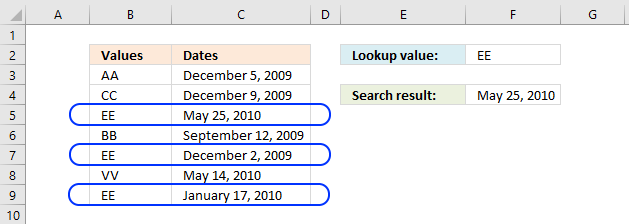The picture above shows you values in column B (B3:B9) and dates in column C (C3:C9). The formula in cell F4 lets you search for value and return the latest date in an adjacent or corresponding column for that value.

#### 1.0.1 Regular formula

Update, 2017-08-15! Added a regular formula.

The following formula is a regular formula, it is somewhat more complicated to understand, however, regular formulas are not prone to errors like array formulas. For example, inexperienced Excel users may easily break array formulas.

Formula in cell F4:

=MAX(INDEX((C3=A8:A14)*B8:B14,))

#### 1.0.2 Array formula

The following formula is an array formula, section 1.2 explains how to enter an array formula.

Array formula in F4:

=MAX(IF(C3=A8:A14, B8:B14))

Section 1.3 explains how the formula above works in great detail.

How to create an array formula

#### 1.0.3 Excel 2019 - regular formula

Formula in cell F4 (Excel 2019):

=MAXIFS(C3:C9, B3:B9,F2)

The MAXIFS function was introduced in Excel 2019, it is entered as a regular formula. You need Excel 2019 or a later Excel version to use this function.

#### 1.0.4 Excel 365 formula

Update, 2021-09-09! Added an Excel 365 formula.

Formula in cell F4 (Excel 365):

=MAX(FILTER(C3:C9,B3:B9=F2))

The FILTER function is a new great function introduced in Excel 365.

### 1.1 Watch a video where I explain the formulas

Recommended article:

Recommended articles

Lookup the nearest date
The image above demonstrates an array formula in cell E4 that searches for the closest date in column A to the […]

### 1.2 How to create an array formula

The formula in 1.0.2 is an array formula and here are the steps to enter an array formula:

1. Double press with left mouse button on cell C5.
2. Copy / Paste above array formula.
3. Press and hold Ctrl + Shift simultaneously.
4. Press Enter.
5. Release all keys.

The formula changes and now begins and ends with a curly bracket, don't enter these characters yourself. They appear automatically.

Recommended article:

Recommended articles

A beginners guide to Excel array formulas
Array formulas allows you to do advanced calculations not possible with regular formulas.

### 1.3 Explaining array formula in cell C5

You can follow along if you select cell C5 and go to tab "Formulas" on the ribbon and then press with left mouse button on "Evaluate Formula" button. Press with left mouse button on "Evaluate" button, shown on the dialog box, to move to next step.

MAX(IF(C3=A8:A14, B8:B14))

#### Step 1 - Find values equal to lookup value

The equal sign is a logical operator that lets you compare value to value, in this case, a comparison value to values is performed. The output is an array of boolean values.

C3=A8:A14

becomes

"EE"={"AA"; "CC"; "EE"; "BB"; "EE"; "VV"; "EE"}

and returns

{FALSE; FALSE; TRUE; FALSE; TRUE; FALSE; TRUE}

Boolean values are either True or False, their numerical equivalents are True - 1 , False - 0 (zero).

#### Step 2 - Convert boolean values to corresponding dates

The IF function returns one value if the logical test is TRUE and another value if the logical test is FALSE.

IF(logical_test, [value_if_true], [value_if_false])

IF(C3=A8:A14, B8:B14)

becomes

IF({FALSE; FALSE; TRUE; FALSE; TRUE; FALSE; TRUE}, B8:B14)

becomes

IF({FALSE; FALSE; TRUE; FALSE; TRUE; FALSE; TRUE} , {40152; 40156; 40323; 40068; 40149; 40312; 40195})

and returns

{FALSE; FALSE; 40323; FALSE; 40149; FALSE; 40195}

#### Step 3 - Return the largest value

The MAX function allows you to calculate the largest number in a cell range. It ignores text, boolean and empty values, however, not error values.

MAX(number1, [number2], ...)

MAX(IF(C3=A8:A14, B8:B14))

becomes

MAX({FALSE; FALSE; 40323; FALSE; 40149; FALSE; 40195})

and returns 40323 formatted as 2010-05-25.

### Get the Excel fileLookup-value-and-return-max-date.xlsx

## 2. Lookup a value and find the most recent date (Pivot Table)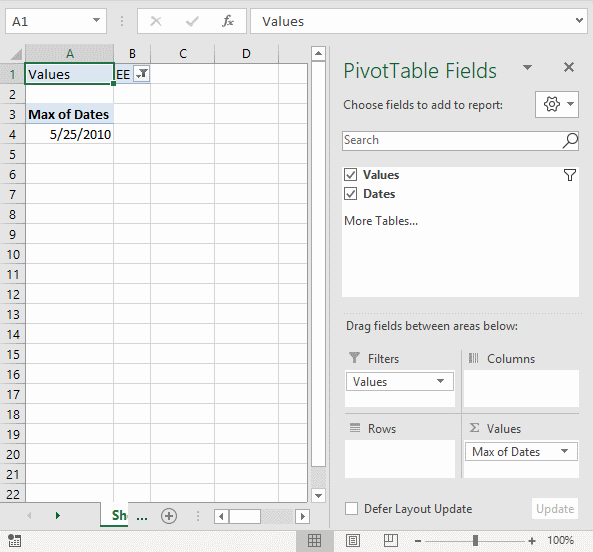The formulas demonstrated in this article may be too slow or taking too much memory if you work with huge amounts of data. The Pivot Table is an excellent option in such cases, it is remarkably fast even with lots of data.

### 2.1 How to set up Pivot Table

1. Select the cell range containing the data.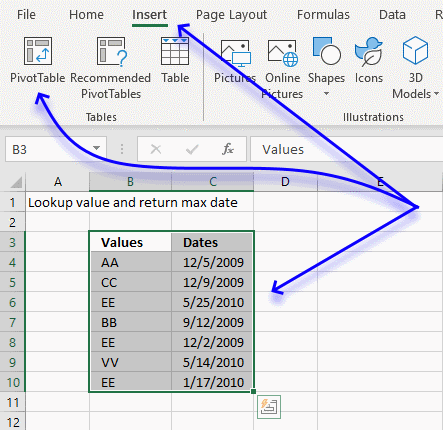2. Go to tab "Insert" on the ribbon.
3. Press with mouse on "Pivot Table" button.
4. A dialog box appears.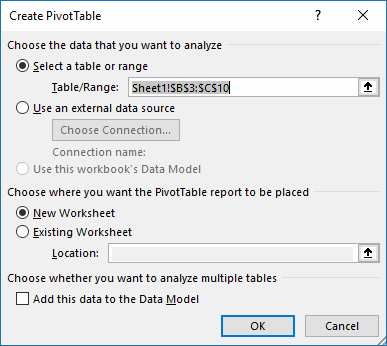I usually place the Pivot Table on a new worksheet so it doesn't hide parts of the data set while filtering etc.
5. Press with left mouse button on OK button.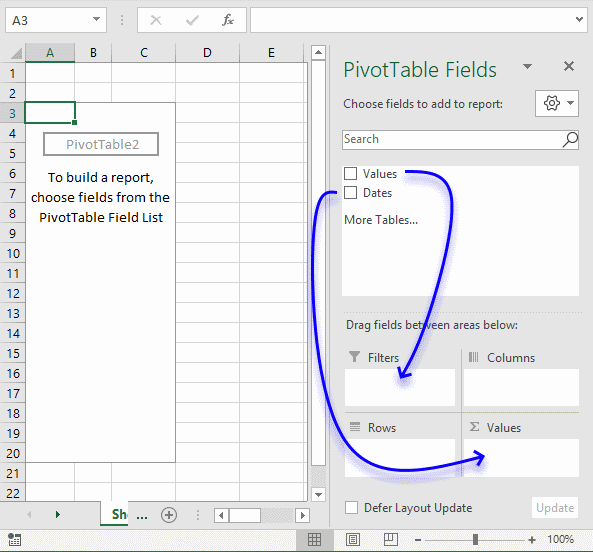1. Press with mouse on Values and drag to Filters field, see blue arrow above.
2. Press with mouse on Dates and drag to Values field.
3. Press with mouse on "Count of Dates".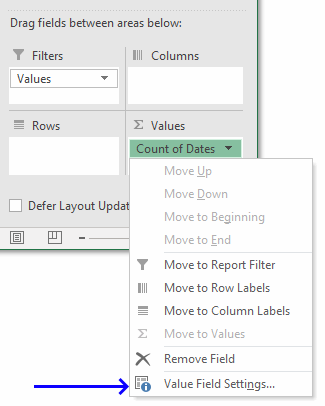4. Press with mouse on "Value Field Settings...".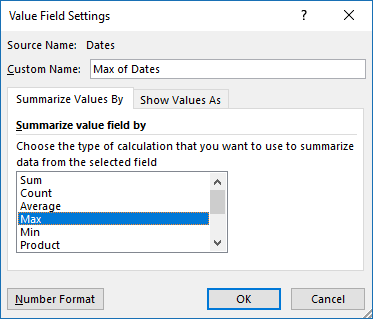5. Press with mouse on "Max" to select it.
6. Press with mouse on "Number Format" button.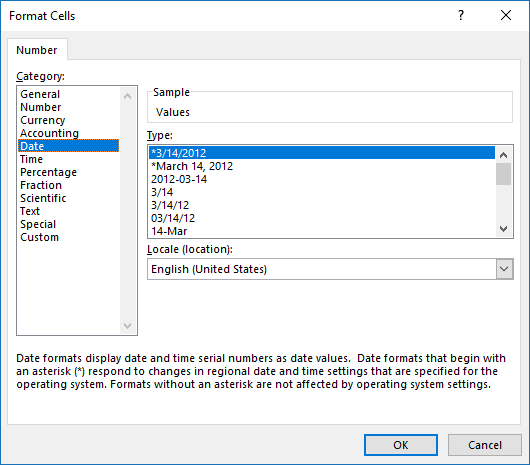7. Press with mouse on category "Date" and select a type.
8. Press with left mouse button on OK button.
9. Press with left mouse button on OK button.Press with mouse on cell B1 to filter the latest date based on the selected value, the image above shows value EE selected and the latest date based on that value is 5/25/2010.

### Get the Excel fileLookup-value-and-return-max-date-pivot-table.xlsx

### 3. Lookup all values and find the most recent date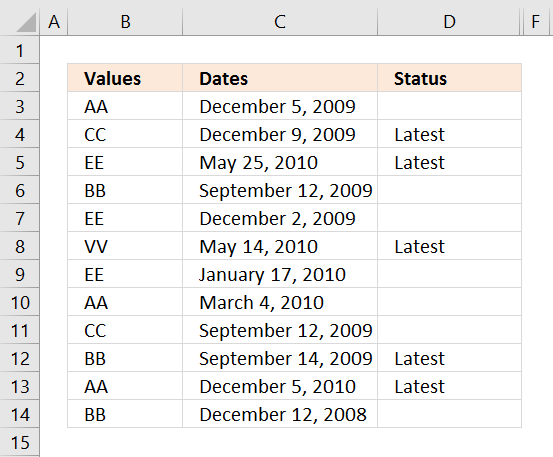The following formula looks in column C for the most recent date based on the value in column B on the same row.

For example, cell B3 contains "AA". Cells B10 and B13 also contain "AA". The corresponding cells on the same row are C3, C10, and C13. They contain these dates "December 9, 2009", "March 4, 2010", and "December 5, 2010".

Cell D3 is not populated with the text "Latest", cell C3 is not the latest date. Cell C13 contains the last date based on the value "AA" in column B on the same row.

The following formula is a regular formula if you prefer that.

Formula in cell D3:

=IF(MAX(INDEX((B3=\$B\$3:\$B\$14)*\$C\$3:\$C\$14, ))=C3, "Latest", "")

The next formula is an array formula, section 1.2 explains how to enter an array formula.

Array formula in cell D3:

=IF(MAX(IF(B3=\$B\$3:\$B\$14, \$C\$3:\$C\$14))=C3, "Latest", "")

The last formula contains an Excel 2016 function, MAXIFS function.

Formula in cell D3:

=IF(MAXIFS(\$C\$3:\$C\$14, \$B\$3:\$B\$14, B3)=C3, "Latest", "")

### 3.1 Watch a video explaining the formula above

3.2 How to copy array formula

1. Copy cell C2
2. Select cell range C3:C8
3. Paste

### 3.3 Explaining formula

This section explains the array formula.

=IF(MAX(IF(B3=\$B\$3:\$B\$14, \$C\$3:\$C\$14))=C3, "Latest", "")

#### Step 1 - Identify cells that meet condition

The equal sign is a logical operator that compares value to value, it returns boolean values True or False.

B3=\$B\$3:\$B\$14

becomes

"AA"={"AA"; "CC"; "EE"; "BB"; "EE"; "VV"; "EE"; "BB"; "CC"; "VV"; "AA"; "CC"}

and returns

{TRUE; FALSE; FALSE; FALSE; FALSE; FALSE; FALSE; FALSE; FALSE; FALSE; TRUE; FALSE}.

#### Step 2 - Replace boolean value with corresponding dates

The IF function returns one value if the logical test is TRUE and another value if the logical test is FALSE.

IF(logical_test, [value_if_true], [value_if_false])

IF(B3=\$B\$3:\$B\$14, \$C\$3:\$C\$14)

becomes

IF({TRUE; FALSE; FALSE; FALSE; FALSE; FALSE; FALSE; FALSE; FALSE; FALSE; TRUE; FALSE}, \$C\$3:\$C\$14)

becomes

IF({TRUE; FALSE; FALSE; FALSE; FALSE; FALSE; FALSE; FALSE; FALSE; FALSE; TRUE; FALSE}, {44554; 44583; 44774; 44725; 44810; 44484; 44771; 44241; 45101; 44218; 44559; 44521})

and returns

{44554; FALSE; FALSE; FALSE; FALSE; FALSE; FALSE; FALSE; FALSE; FALSE; 44559; FALSE}

#### Step 3 - Extract latest date

The MAX function allows you to calculate the largest number in a cell range. It ignores text, boolean and empty values, however, not error values.

MAX(number1, [number2], ...)

MAX(IF(B3=\$B\$3:\$B\$14, \$C\$3:\$C\$14))

becomes

MAX({44554; FALSE; FALSE; FALSE; FALSE; FALSE; FALSE; FALSE; FALSE; FALSE; 44559; FALSE})

and returns 44559.

#### Step 4 - Compare with corresponding date on the same row

MAX(IF(B3=\$B\$3:\$B\$14, \$C\$3:\$C\$14))=C3

becomes

44559=44554

and returns False.

#### Step 5 - Return "Latest" if True

IF(MAX(IF(B3=\$B\$3:\$B\$14, \$C\$3:\$C\$14))=C3, "Latest", "")

becomes

IF(FALSE , "Latest", "")

and returns "" nothing in cell D3.

### Get the Excel fileLookup-all-values-and-find-the-latest-date.xlsx

## 4. Lookup and find the most recent date using multiple conditions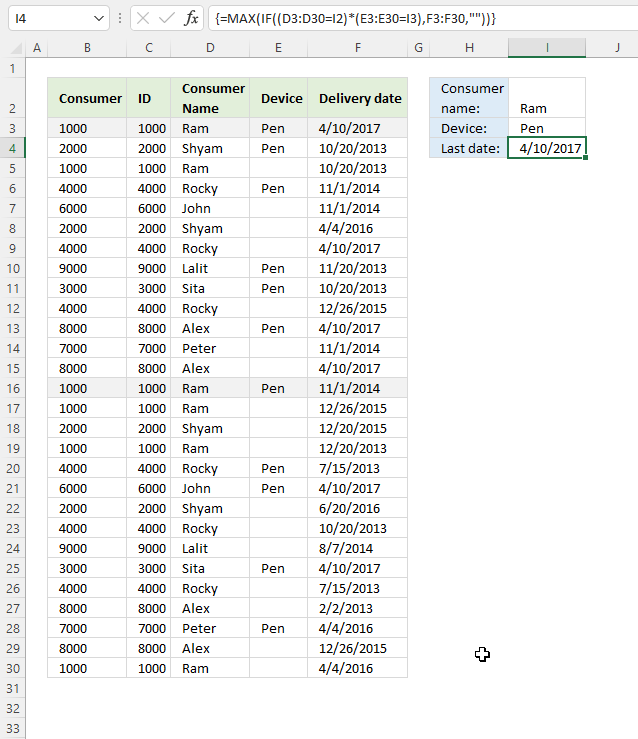This example demonstrates a formula that uses two conditions to filter the latest date.

For example, the first condition is "Ram" and the second condition is "Pen". The formula calculates the latest date from column E if both conditions are true on the same row.

The first condition and the second condition are both found on rows 2 and 15, they are highlighted in the image above. The corresponding dates are 4/10/2017 and 11/1/2014, the latest date is 4/10/2017 which is returned in cell H3.

Formula in cell H3:

=MAX(INDEX((D3:D30=I2)*(E3:E30=I3)*F3:F30,))

Array formula in cell H3:

=MAX(IF((D3:D30=I2)*(E3:E30=I3),F3:F30,""))

How to create an array formula

### 4.1 Explaining array formula

#### Step 1 - First condition

The equal sign is a logical operator that compares value to value, it returns boolean values True or False.

D3:D30=I2

becomes

{"Ram"; "Shyam"; "Ram"; "Rocky"; "John"; "Shyam"; "Rocky"; "Lalit"; "Sita"; "Rocky"; "Alex"; "Peter"; "Alex"; "Ram"; "Ram"; "Shyam"; "Ram"; "Rocky"; "John"; "Shyam"; "Rocky"; "Lalit"; "Sita"; "Rocky"; "Alex"; "Peter"; "Alex"; "Ram"}="Ram"

and returns

{TRUE; FALSE; TRUE; FALSE; FALSE; FALSE; FALSE; FALSE; FALSE; FALSE; FALSE; FALSE; FALSE; TRUE; TRUE; FALSE; TRUE; FALSE; FALSE; FALSE; FALSE; FALSE; FALSE; FALSE; FALSE; FALSE; FALSE; TRUE}.

#### Step 2 - Second condition

E3:E30=I3

becomes

{"Pen"; "Pen"; 0; "Pen"; 0; 0; 0; "Pen"; "Pen"; 0; "Pen"; 0; 0; "Pen"; 0; 0; 0; "Pen"; "Pen"; 0; 0; 0; "Pen"; 0; 0; "Pen"; 0; 0}="Pen"

and returns

{TRUE; TRUE; FALSE; TRUE; FALSE; FALSE; FALSE; TRUE; TRUE; FALSE; TRUE; FALSE; FALSE; TRUE; FALSE; FALSE; FALSE; TRUE; TRUE; FALSE; FALSE; FALSE; TRUE; FALSE; FALSE; TRUE; FALSE; FALSE}

#### Step 3 - Multiply arrays - AND logic

The asterisk lets you multiply arrays, this performs AND-logic meaning if both values are TRUE then return TRUE or their numerical equivalent 1. All other combinations return FALSE or 0 (zero).

The parenthesis lets you control the order of calculation, we want to compare values before we multiply the arrays.

(D3:D30=I2)*(E3:E30=I3)

becomes

({"Ram"; "Shyam"; "Ram"; "Rocky"; "John"; "Shyam"; "Rocky"; "Lalit"; "Sita"; "Rocky"; "Alex"; "Peter"; "Alex"; "Ram"; "Ram"; "Shyam"; "Ram"; "Rocky"; "John"; "Shyam"; "Rocky"; "Lalit"; "Sita"; "Rocky"; "Alex"; "Peter"; "Alex"; "Ram"}="Ram")*({"Pen"; "Pen"; 0; "Pen"; 0; 0; 0; "Pen"; "Pen"; 0; "Pen"; 0; 0; "Pen"; 0; 0; 0; "Pen"; "Pen"; 0; 0; 0; "Pen"; 0; 0; "Pen"; 0; 0}="Pen")

becomes

{TRUE; FALSE; TRUE; FALSE; FALSE; FALSE; FALSE; FALSE; FALSE; FALSE; FALSE; FALSE; FALSE; TRUE; TRUE; FALSE; TRUE; FALSE; FALSE; FALSE; FALSE; FALSE; FALSE; FALSE; FALSE; FALSE; FALSE; TRUE}*{TRUE; TRUE; FALSE; TRUE; FALSE; FALSE; FALSE; TRUE; TRUE; FALSE; TRUE; FALSE; FALSE; TRUE; FALSE; FALSE; FALSE; TRUE; TRUE; FALSE; FALSE; FALSE; TRUE; FALSE; FALSE; TRUE; FALSE; FALSE}

and returns

{1; 0; 0; 0; 0; 0; 0; 0; 0; 0; 0; 0; 0; 1; 0; 0; 0; 0; 0; 0; 0; 0; 0; 0; 0; 0; 0; 0}.

#### Step 4 - Replace boolean values with corresponding dates

The IF function returns one value if the logical test is TRUE and another value if the logical test is FALSE.

IF(logical_test, [value_if_true], [value_if_false])

IF((D3:D30=I2)*(E3:E30=I3),F3:F30,"")

becomes

IF({1; 0; 0; 0; 0; 0; 0; 0; 0; 0; 0; 0; 0; 1; 0; 0; 0; 0; 0; 0; 0; 0; 0; 0; 0; 0; 0; 0}, F3:F30, "")

becomes

IF({1; 0; 0; 0; 0; 0; 0; 0; 0; 0; 0; 0; 0; 1; 0; 0; 0; 0; 0; 0; 0; 0; 0; 0; 0; 0; 0; 0}, {42835; 41567; 41567; 41944; 41944; 42464; 42835; 41598; 41567; 42364; 42835; 41944; 42835; 41944; 42364; 42358; 41628; 41470; 42835; 42541; 41567; 41858; 42835; 41470; 41307; 42464; 42364; 42464}, "")

and returns

{42835; ""; ""; ""; ""; ""; ""; ""; ""; ""; ""; ""; ""; 41944; ""; ""; ""; ""; ""; ""; ""; ""; ""; ""; ""; ""; ""; ""}.

#### Step 5 - Extract latest date

The MAX function allows you to calculate the largest number in a cell range. It ignores text, boolean and empty values, however, not error values.

MAX(number1, [number2], ...)

MAX(IF((D3:D30=I2)*(E3:E30=I3),F3:F30,""))

becomes

MAX({42835; ""; ""; ""; ""; ""; ""; ""; ""; ""; ""; ""; ""; 41944; ""; ""; ""; ""; ""; ""; ""; ""; ""; ""; ""; ""; ""; ""})

and returns 42835 (4/10/2017) which is the largest number in the array.

### Get the Excel fileLookup-and-find-last-date-using-multiple-conditions.xlsx

## 5. Lookup and find the most recent date on multiple sheets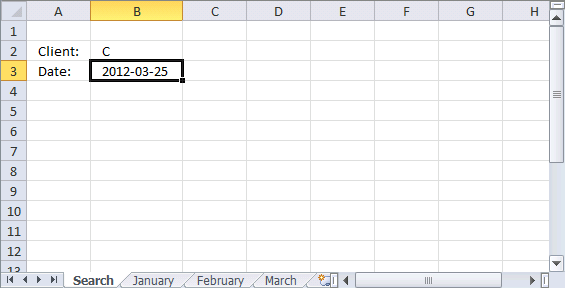The picture above shows a workbook with 4 worksheets named "Search", January, February, and March. The formula in cell B3 looks for the latest date in all three worksheets using the condition in cell B2.

Unfortunately, it is not possible to use 3d references with IF functions. The formula contains three IF functions to make it possible to search all three worksheets.

Array formula in cell B3:

=MAX(IF(B2=January!\$B\$2:\$B\$10, January!\$A\$2:\$A\$10, ""), IF(B2=February!\$B\$2:\$B\$10, February!\$A\$2:\$A\$10, ""), IF(B2=March!\$B\$2:\$B\$10, March!\$A\$2:\$A\$10, ""))

Update! Excel 365 dynamic array formula:

=MAX(FILTER(VSTACK(January:March!A2:A10), VSTACK(January:March!B2:B10)=B2))

### 5.1 Explaining array formula

#### Step 1 - Logical test

The equal sign is a logical operator that lets you compare value to value. It is also possible to compare a value to multiple values in the same calculations, the result is an array of boolean values TRUE or FALSE.

B2=January!\$B\$2:\$B\$10

becomes

"C"={"A"; "B"; "C"; "A"; "B"; "C"; "A"; "B"; "C"}

and returns

{FALSE; FALSE; TRUE; FALSE; FALSE; TRUE; FALSE; FALSE; TRUE}.

#### Step 2 - Filter dates from the worksheet named January

The IF function returns one value if the logical test is TRUE and another value if the logical test is FALSE.

Function syntax: IF(logical_test, [value_if_true], [value_if_false])

IF(B2=January!\$B\$2:\$B\$10, January!\$A\$2:\$A\$10, "")

becomes

IF({FALSE; FALSE; TRUE; FALSE; FALSE; TRUE; FALSE; FALSE; TRUE}, {40935; 40912; 40929; 40910; 40932; 40920; 40935; 40914; 40914}, "")

and returns

{""; ""; 40929; ""; ""; 40920; ""; ""; 40914}.

#### Step 3 - Logical test

B2=February!\$B\$2:\$B\$10

becomes

"C"={"A"; "B"; "C"; "A"; "B"; "C"; "A"; "B"; "C"}

and returns

{FALSE; FALSE; TRUE; FALSE; FALSE; TRUE; FALSE; FALSE; TRUE}

#### Step 4 - Filter dates from the worksheet named February

The IF function returns one value if the logical test is TRUE and another value if the logical test is FALSE.

Function syntax: IF(logical_test, [value_if_true], [value_if_false])

IF(B2=February!\$B\$2:\$B\$10, February!\$A\$2:\$A\$10, "")

becomes

IF({FALSE; FALSE; TRUE; FALSE; FALSE; TRUE; FALSE; FALSE; TRUE}, {40963; 40944; 40962; 40967; 40957; 40950; 40958; 40941; 40966}, "")

and returns

{""; ""; 40962; ""; ""; 40950; ""; ""; 40966}.

#### Step 5 - Logical test

B2=March!\$B\$2:\$B\$10

becomes

"C"={"D"; "B"; "C"; "D"; "B"; "C"; "D"; "B"; "C"}

and returns

{FALSE; FALSE; TRUE; FALSE; FALSE; TRUE; FALSE; FALSE; TRUE}

#### Step 6 - Filter dates from worksheet named March

The IF function returns one value if the logical test is TRUE and another value if the logical test is FALSE.

Function syntax: IF(logical_test, [value_if_true], [value_if_false])

IF(B2=March!\$B\$2:\$B\$10, March!\$A\$2:\$A\$10, "")

becomes

IF({FALSE; FALSE; TRUE; FALSE; FALSE; TRUE; FALSE; FALSE; TRUE}, {40978; 40988; 40993; 40973; 40983; 40981; 40993; 40978; 40970}, "")

and returns

{""; ""; 40993; ""; ""; 40981; ""; ""; 40970}.

#### Step 7 - Calculate largest number from three arrays

The MAX function calculate the largest number in a cell range.

Function syntax: MAX(number1, [number2], ...)

MAX(IF(B2=January!\$B\$2:\$B\$10, January!\$A\$2:\$A\$10, ""), IF(B2=February!\$B\$2:\$B\$10, February!\$A\$2:\$A\$10, ""), IF(B2=March!\$B\$2:\$B\$10, March!\$A\$2:\$A\$10, ""))

becomes

MAX({""; ""; 40929; ""; ""; 40920; ""; ""; 40914}, {""; ""; 40962; ""; ""; 40950; ""; ""; 40966}, {""; ""; 40993; ""; ""; 40981; ""; ""; 40970})

and returns

40993. (3/25/2012)

### 5.2 Lookup and find the most recent date on multiple sheets - Excel 365Excel 365 dynamic array formula in cell B3:

=MAX(FILTER(VSTACK(January:March!A2:A10),VSTACK(January:March!B2:B10)=B2))

### 5.3 Explaining Excel 365 dynamic array formula

#### Step 1 - Create 3D reference

Here is how to create a 3D reference in cell B3:

1. Select cell B3.
2. Type the equal sign (=) to start creating the formula.
3. Select cell range A2:A10 from the first worksheet (January).
4. Press and hold CTRL key.
5. Press with left mouse button on the sheet tab of the last worksheet (March) that you want to reference.

Note: To create a 3D reference, all the worksheets must be in the same workbook.

This is what the cell reference should look like in cell B3.

=January:March!A2:A10

#### Step 2 - Merge values from given cell ranges

The VSTACK function combines cell ranges or arrays. Joins data to the first blank cell at the bottom of a cell range or array (vertical stacking)

Function syntax: VSTACK(array1,[array2],...)

VSTACK(January:March!A2:A10)

becomes

VSTACK({40935; 40912; 40929; 40910; 40932; 40920; 40935; 40914; 40914}, {40963; 40944; 40962; 40967; 40957; 40950; 40958; 40941; 40966}, {40978; 40988; 40993; 40973; 40983; 40981; 40993; 40978; 40970})

and returns

{40935; 40912; 40929; 40910; 40932; 40920; 40935; 40914; 40914; 40963; 40944; 40962; 40967; 40957; 40950; 40958; 40941; 40966; 40978; 40988; 40993; 40973; 40983; 40981; 40993; 40978; 40970}

#### Step 3 - Create a logical array containing TRUE or FALSE based on the condition specified in cell B2

This part of the formula merges the values in cell ranges B2:B10 in worksheets from January to March.

The equal sign compares the values to the specified condition in cell B2, the result is a boolean array containing TRUE or FALSE.

VSTACK(January:March!B2:B10)=B2

becomes

{"A"; "B"; "C"; "A"; "B"; "C"; "A"; "B"; "C"; "A"; "B"; "C"; "A"; "B"; "C"; "A"; "B"; "C"; "D"; "B"; "C"; "D"; "B"; "C"; "D"; "B"; "C"}="C"

and returns

{FALSE; FALSE; TRUE; FALSE; FALSE; TRUE; FALSE; FALSE; TRUE; FALSE; FALSE; TRUE; FALSE; FALSE; TRUE; FALSE; FALSE; TRUE; FALSE; FALSE; TRUE; FALSE; FALSE; TRUE; FALSE; FALSE; TRUE}.

The semicolon ; is a row delimiting character in Excel arrays.

#### Step 3 - Filter dates based on the condition specified in cell B2

The FILTER function extracts values/rows based on a condition or criteria.

Function syntax: FILTER(array, include, [if_empty])

FILTER(VSTACK(January:March!A2:A10),VSTACK(January:March!B2:B10)=B2)

becomes

FILTER({40935; 40912; 40929; 40910; 40932; 40920; 40935; 40914; 40914; 40963; 40944; 40962; 40967; 40957; 40950; 40958; 40941; 40966; 40978; 40988; 40993; 40973; 40983; 40981; 40993; 40978; 40970}, {FALSE; FALSE; TRUE; FALSE; FALSE; TRUE; FALSE; FALSE; TRUE; FALSE; FALSE; TRUE; FALSE; FALSE; TRUE; FALSE; FALSE; TRUE; FALSE; FALSE; TRUE; FALSE; FALSE; TRUE; FALSE; FALSE; TRUE})

and returns

{40929; 40920; 40914; 40962; 40950; 40966; 40993; 40981; 40970}.

#### Step 4 - Get the latest date

The MAX function calculate the largest number in a cell range.

Function syntax: MAX(number1, [number2], ...)

MAX(FILTER(VSTACK(January:March!A2:A10),VSTACK(January:March!B2:B10)=B2))

becomes

MAX({40929; 40920; 40914; 40962; 40950; 40966; 40993; 40981; 40970})

and returns

40993. 40993 represents the date 3/25/2012 in Excel.

### Get the Excel fileFind-last-appointment-date.xlsx

## 6. Lookup and find the most recent date, return the corresponding value on the same row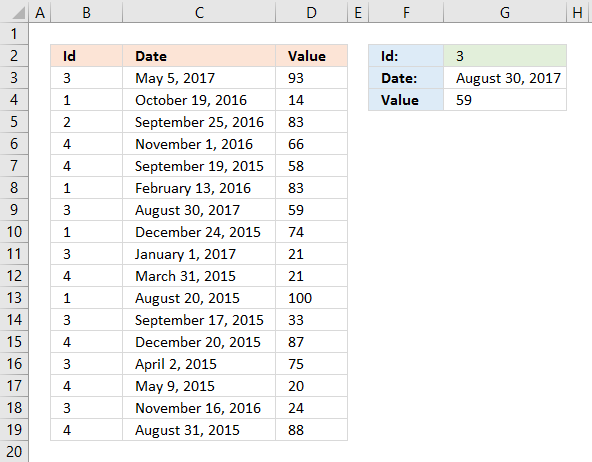Enter a condition in cell G3, the formula extracts the latest date. Another formula in cell G3 gets the corresponding value on the same row from column D.

Array formula in cell G3:

=MAX((B3:B19=G2)*C3:C19)

If you prefer a regular formula in G3:

=MAX(INDEX((B3:B19=G2)*C3:C19,))

Update! New array formula in cell G4:

=INDEX(D3:D19,MATCH(1,(B3:B19=G2)*(C3:C19=G3),0))

Excel 365 formula in cell G4:

=FILTER(D3:D19,(B3:B19=G2)*(G3=C3:C19))

Old Formula in cell G4:

=INDEX(\$D\$3:\$D\$19, SUMPRODUCT((B3:B19=G2)*(G3=C3:C19)*MATCH(ROW(B3:B19), ROW(B3:B19))))

### 6.2 Explaining formula in cell G4

Formulas in cell G3 are explained in section 1 above.

#### Step 1 - First condition

The equal sign is a logical operator that lets you compare value to value or in this case value to an array of values. The result is an array of boolean values TRUE and FALSE.

This logical expression compares the condition specified in cell G2 to the values in B3:B19.

B3:B19=G2

becomes

{3; 1; 2; 4; 4; 1; 3; 1; 3; 4; 1; 3; 4; 3; 4; 3; 4}=3

and returns

{TRUE; FALSE; FALSE; FALSE; FALSE; FALSE; TRUE; FALSE; TRUE; FALSE; FALSE; TRUE; FALSE; TRUE; FALSE; TRUE; FALSE}.

#### Step 2 - Second condition

C3:C19=G3

becomes

{42860; 42662; 42638; 42675; 42266; 42413; 42977; 42362; 42736; 42094; 42236; 42264; 42358; 42096; 42133; 42690; 42247}=42977

and returns

{FALSE; FALSE; FALSE; FALSE; FALSE; FALSE; TRUE; FALSE; FALSE; FALSE; FALSE; FALSE; FALSE; FALSE; FALSE; FALSE; FALSE}

#### Step 3 - Multiply arrays to perform AND logic

The asterisk character lets you multiply numbers in an Excel formula. The parentheses lets you control the order of operation.

(B3:B19=G2)*(C3:C19=G3)

becomes

({TRUE; FALSE; FALSE; FALSE; FALSE; FALSE; TRUE; FALSE; TRUE; FALSE; FALSE; TRUE; FALSE; TRUE; FALSE; TRUE; FALSE})*({FALSE; FALSE; FALSE; FALSE; FALSE; FALSE; TRUE; FALSE; FALSE; FALSE; FALSE; FALSE; FALSE; FALSE; FALSE; FALSE; FALSE})

and returns

{0; 0; 0; 0; 0; 0; 1; 0; 0; 0; 0; 0; 0; 0; 0; 0; 0}

#### Step 4 - Find the relative position

The MATCH function returns the relative position of an item in an array that matches a specified value in a specific order.

Function syntax: MATCH(lookup_value, lookup_array, [match_type])

MATCH(1,(B3:B19=G2)*(C3:C19=G3),0)

becomes

MATCH(1,{0; 0; 0; 0; 0; 0; 1; 0; 0; 0; 0; 0; 0; 0; 0; 0; 0},0)

and returns 7.

Number 1 is in position 7 in the array.

#### Step 5 - Get value based on the relative position

The INDEX function returns a value or reference from a cell range or array, you specify which value based on a row and column number.

Function syntax: INDEX(array, [row_num], [column_num])

INDEX(D3:D19,MATCH(1,(B3:B19=G2)*(C3:C19=G3),0))

becomes

INDEX(D3:D19,7)

and returns the value in cell D9 which is 59.